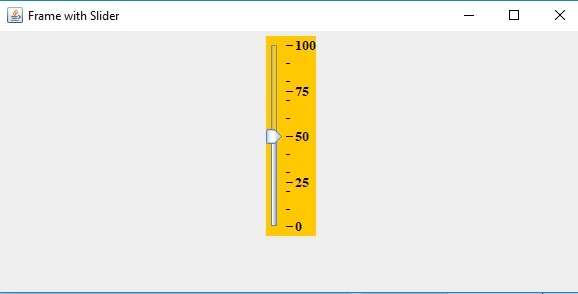# Create a vertical slider with custom min, max, and initial value in Java

While creating vertical slider, we can set custom values as well. Let us take three integer variable and set the int values for min, max as well as the initial value of the slider −

int val = 50;
int min = 0;
int max = 100;

Set it to the slider while creating a new slider. Here, we have set the constant to be VERTICAL, since we are creating a vertical slider −

JSlider slider = new JSlider(JSlider.VERTICAL,min, max, val);

The following is an example to create a vertical slider with custom values −

## Example

package my;
import java.awt.Color;
import java.awt.Font;
import javax.swing.JFrame;
import javax.swing.JPanel;
import javax.swing.JSlider;
import javax.swing.WindowConstants;
public class SwingDemo {
public static void main(String[] args) {
JFrame frame = new JFrame("Frame with Slider");
int val = 50;
int min = 0;
int max = 100;
JSlider slider = new JSlider(JSlider.VERTICAL,min, max, val);
slider.setMinorTickSpacing(10);
slider.setMajorTickSpacing(25);
slider.setPaintTicks(true);
slider.setPaintLabels(true);
slider.setBackground(Color.ORANGE);
slider.setForeground(Color.black);
slider.setSnapToTicks(true);
System.out.println("Snapping to tick marks? = "+slider.getSnapToTicks());
Font font = new Font("Serif", Font.BOLD, 13);
slider.setFont(font);
JPanel panel = new JPanel();
}